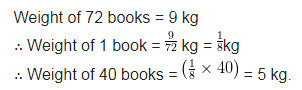# The weight of 72 books is 9 kg. What is the weight of 40 such books?

The weight of 72 books is 9 kg. What is the weight of 40 such books?